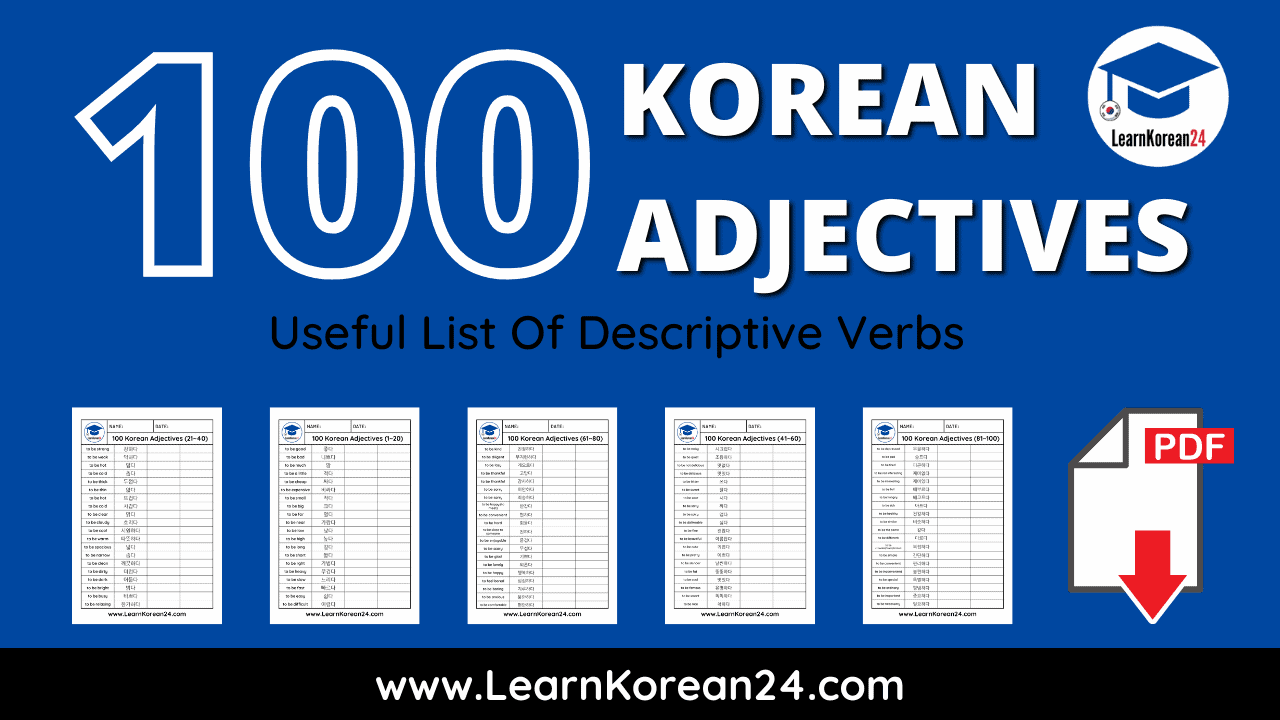Learning many Korean adjectives is a great way to expand your vocabulary and Korean language skills as it will allow you to make many more varied sentences. To help you learn many Korean adjectives, we have put together this Korean adjective list with 100 useful Korean descriptive verbs.

Before we look at the list of Korean adjectives, it is important to note that you may also hear Korean adjectives referred to as ‘descriptive verbs’. This is because, in Korean, the base form of adjectives are written in the infinitive verb form. For example, when you look up the adjective ‘hot’ in a Korean dictionary, it will be listed in the infinitive verb form which is 덥다 (to be hot). To use a Korean adjective in a sentence, you must take this infinitive form and conjugate it into the relevant tense.

All the Korean adjectives (descriptive verbs) on this page are listed in the infinitive form for you to learn. Below you will find a list of 100 Korean adjectives divided into sections of 20. You’ll also be able to download this list of Korean adjectives as a printable PDF after each section. If you would like to check how to pronounce these Korean adjectives, check out the video at the bottom of the page.

Related: 100 Korean Verbs For Beginners

Here is a list of 100 useful Korean adjectives for you to learn:

• 좋다 = to be good
• 나쁘다 = to be bad
• 많다 = to be much
• 적다 = to be a little
• 싸다 = to be cheap
• 비싸다 = to be expensive
• 작다 = to be small
• 크다 = to be big
• 멀다 = to be far
• 가깝다 = to be near
• 낮다 = to be low
• 높다 = to be high
• 길다 = to be long
• 짧다 = to be short
• 가볍다 = to be light
• 무겁다 = to be heavy
• 느리다 = to be slow
• 빠르다 = to be fast
• 쉽다 = to be easy
• 어렵다 = to be difficult

• 강하다 = to be strong
• 약하다 = to be weak
• 덥다 = to be hot
• 춥다 = to be cold
• 두껍다 = to be thick
• 얇다 = to be thin
• 뜨겁다 = to be hot
• 차갑다 = to be cold
• 맑다 = to be clear
• 흐리다 = to be cloudy
• 시원하다 = to be cool
• 따뜻하다 = to be warm
• 넓다 = to be spacious
• 좁다 = to be narrow
• 깨끗하다 = to be clean
• 더럽다 = to be dirty
• 어둡다 = to be dark
• 밝다 = to be bright
• 바쁘다 = to be busy
• 한가하다 = to be relaxing

• 시끄럽다 = to be noisy
• 조용하다 = to be quiet
• 맛없다 = to be not delicious
• 맛있다 = to be delicious
• 쓰다 = to be bitter
• 달다 = to be sweet
• 시다 = to be sour
• 짜다 = to be salty
• 맵다 = to be spicy
• 싫다 = to be dislikeable
• 괜찮다 = to be fine
• 아름답다 = to be beautiful
• 귀엽다 = to be cute
• 예쁘다 = to be pretty
• 날씬하다 = to be slender
• 뚱뚱하다 = to be fat
• 멋있다 = to be cool (appearance)
• 유명하다 = to be famous
• 똑똑하다 = to be smart
• 착하다 = to be nice (personality)

• 친절하다 = to be kind
• 부지런하다 = to be diligent
• 게으르다 = to be lazy
• 고맙다 = to be thankful (less formal)
• 감사하다 = to be thankful (formal)
• 미안하다 = to be sorry (less formal)
• 죄송하다 = to be sorry (formal)
• 반갑다 = to be happy(to meet)
• 편하다 = to be easy/convenient
• 힘들다 = to be hard
• 친하다 = to be close (to someone)
• 즐겁다 = to be enjoyable
• 무섭다 = to be scary
• 기쁘다 = to be glad
• 외롭다 = to be lonely
• 행복하다 = to be happy
• 심심하다 = to feel bored
• 지루하다 = to be boring
• 불안하다 = to be anxious
• 편안하다 = to be comfortable

• 우울하다 = to be depressed
• 슬프다 = to be sad
• 피곤하다 = to be tired
• 재미없다 = to be not interesting
• 재미있다 = to be interesting
• 배부르다 = to be full
• 배고프다 = to be hungry
• 아프다 = to be sick
• 건강하다 = to be healthy
• 비슷하다 = to be similar
• 같다 = to be the same
• 다르다 = to be different
• 복잡하다 = to be crowded, to be complicated
• 간단하다 = to be simple
• 편리하다 = to be convenient
• 불편하다 = to be inconvenient
• 특별하다 = to be special
• 평범하다 = to be ordinary
• 중요하다 = to be important
• 필요하다 = to be necessary